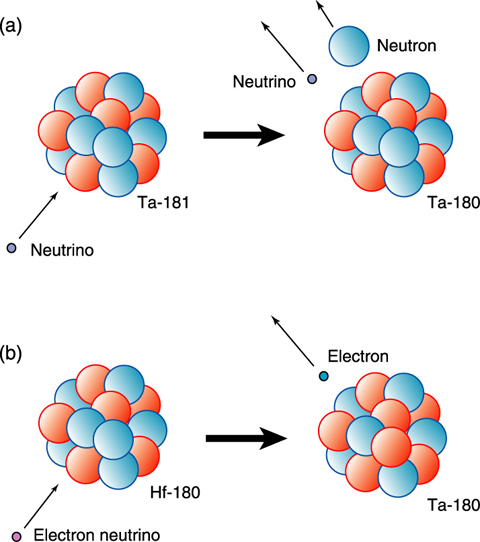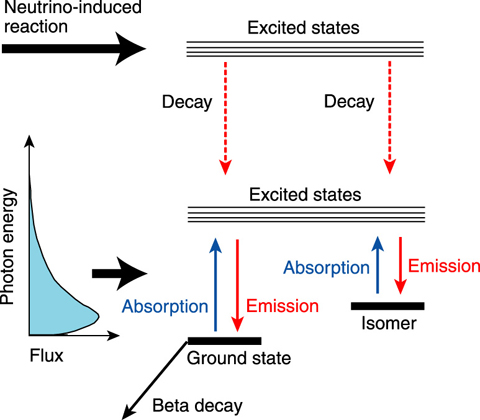# 4-6 Supernova Neutrino-Process Nucleosynthesis

## －Astrophysical Origin of 180Ta, the Rarest Nuclide in the Solar System－Fig.4-12　Schematic view of production of 180Ta by neutrino transitions induced reactions

180Ta is produced by (a) a neutrino-induced reaction in which a neutron is evaporated and by (b) a charge exchange reaction where a neutron is changed to a proton.Fig.4-13　Schematic views of nuclear structure of 180Ta and between the ground state and the isomer

The neutrino-induced reaction produces the ground state and isomer in 180Ta. These two states are linked by an interaction of photon absorption and emission.

The astrophysical origins of about 290 nuclides have been studied but the nucleosynthesis of 180Ta has remained an unsolved problem. A unique feature of this nuclide is that it is the rarest in the solar system. Over the past 30 years many processes such as rapid neutron capture reactions in supernova explosions, photon-induced reactions in supernovae, and galactic cosmic ray production have been proposed as the origin of 180Ta. However, previous theoretical calculations based on these proposed models could not reproduce the solar abundance of 180Ta.

Neutrino-induced reactions in supernovae have been proposed as the origin of 180Ta. A proto-neutron star formed in the early stage of a supernova emits huge neutrinos; these neutrinos produce 180Ta by neutrino-nucleus reactions on 180Hf and 181Ta in outer layers (Fig.4-12). However, the theoretical calculation overproduces the solar abundance of 180Ta. This overproduction problem originates from the fact that previous models could not calculate a meta-stable isomer residual ratio using a time-dependent model. The nuclear structure of 180Ta has a feature that the ground state beta decays with a half-life of about 8 hours, whereas the isomer at 77 keV is meta-stable.

In extremely high temperature environments in supernovae, the ground state is linked through highly excited states by (gamma, gamma’) reactions. The transition probability from the ground state (isomer) to the isomer (ground state) is a function of temperature. In supernova explosions, the temperature of the neutrino process layers becomes higher than 109 K and suddenly decreases in a time scale of several seconds.

For our purposes we can model the excited-state structure as consisting of two sets of nuclear states: (1) the ground state structure, which consists of the ground state plus the excited states with strong transitions to the ground state; and (2) the analogous isomeric structure (Fig.4-13). The time-dependent evolution of the residual isomeric ratio is developed using the two structure model. The resulting isomeric residual population ratio isomer/(ground state + isomer) is found to be 0.39.

We also found that the final result, namely that the isomer residual ratio is 0.39, is nearly independent of a number of astrophysical parameters such as the progenitor mass, the supernova explosion energy, the neutrino energy spectrum, or the peak temperature of the nucleosynthesis environment. Using the ratio of 0.39, we found that the solar abundance of 180Ta can be reproduced by the neutrino process with average electron neutrino energy of 12 MeV.

| | | | |